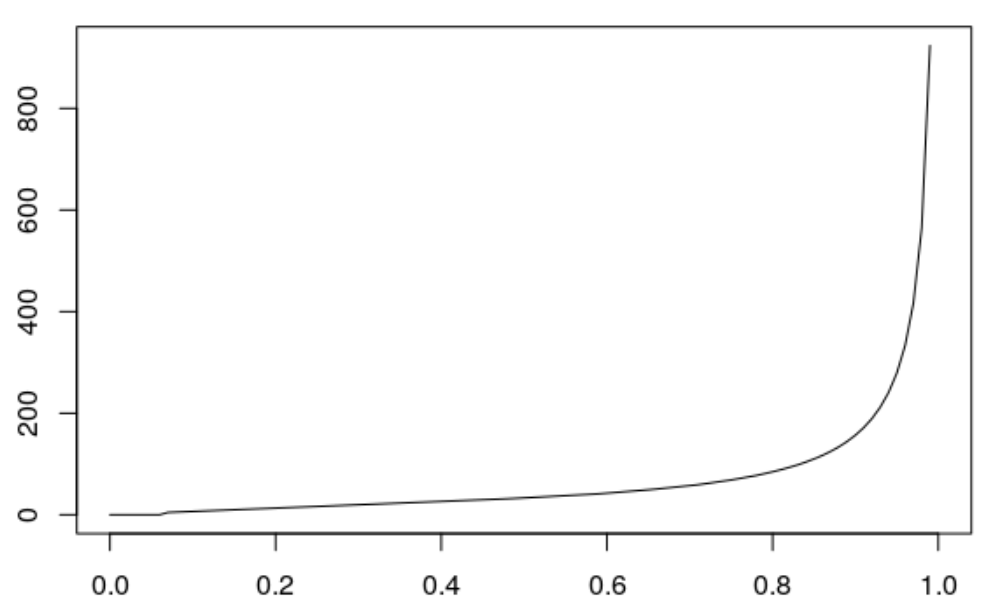Want to share your content on R-bloggers? click here if you have a blog, or here if you don't.

Usually, to illustrate the difference between S3 and S4 classes in R, I mention glm (from base) and vglm (from VGAM) that provide similar outputs, but one is based on S3 codes, while the second one is based on S4 codes. Another way to illustrate is to manipulate distributions.

Consider the case where we want to sum (independent) random variables. For instance two lognormal distribution. Let us try to compute the median of the sum.

The distribution function of the sum of two independent (positive) random variables is $$F_{S_2}(x)=\int_0^x F_{X_1}(x-y)dF_{X_2}(x)$$

pSum2 = function(x) integrate(function(y)
plnorm(x-y,1,2)*dlnorm(y,2,1),0,x)$value Let us visualize that cumulative distribution function vx=seq(0.1,50,by=.1) vy=Vectorize(pSum2)(vx) plot(vx,vy,type="l",ylim=c(0,1)) abline(h=.5,lty=2)Let us find an upper bound to compute (in a decent time) quantiles pSum2(350)  0.99195 and then use the uniroot function to inverse that function qSum = function(u) uniroot(function(x) Vectorize(pSum2)(x)-u, interval=c(0,350))$root
vu=seq(.01,.99,by=.01)
vv=Vectorize(qSum)(vu)The median is here

qSum(.5)
 14.155

Why not consider the sum of three (independent) distributions ? Its cumulative distribution function can be writen using our previous function $$F_{S_3}(x)=\int_0^x F_{S_2}(x-y)dF_{X_3}(x)$$

pSum3 = function(x) integrate(function(y)
pSum2(x-y)*dlnorm(y,2,2),0,x)$value If we look at some values we good pSum3(4)  0.015624 pSum3(5) Error in integrate(function(y) plnorm(x - y, 1, 2) * dlnorm(y, 2, 1), : maximum number of subdivisions reached So obviously, there are computational issues here. Let us consider the following alternative expression $$F_{S_3}(x)=\int_0^x F_{X_3}(x-y)dF_{S_2}(x)$$. Of course, it is necessary here to compute the density of the sum of two variables dSum2 = function(x) integrate(function(y) dlnorm(x-y,1,2)*dlnorm(y,2,1),0,x)$value
pSum3 = function(x) integrate(function(y)
dlnorm(x-y,2,2)*dSum2(y),0,x)\$value

Again, let us compute some values

pSum3(4)
 0.0090285
pSum3(5)
 0.01186

This one seems to work quite well. But it is just an illusion.

pSum3(9)
Error in integrate(function(y) dlnorm(x - y, 1, 2) *
dlnorm(y, 2, 1),  :
maximum number of subdivisions reached

Clearly, with those S3-type functions, it wlll be complicated to run computations with 3 variables, or more.

Let us consider distributions in the S4-type format of the following package

library(distr)
X1 = Lnorm(mean=1,sd=2)
X2 = Lnorm(mean=2,sd=1)
S2 = X1+X2

To compute the median, we simply have to use

distr::q(S2)(.5)
 14.719

We can also visualize it easily

plot(q(S2))which looks (very) close to what we got, manually.  But here, it is also possible to work with the sum of 3 (independent) random variables

X3 = Lnorm(mean=2,sd=2)
S3 = X1+X2+X3

To compute the median, use

distr::q(S3)(.5)
 33.208

The function is here

plot(q(S3))